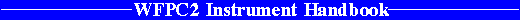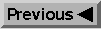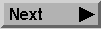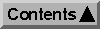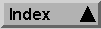System Throughput and SNR / Exposure Time Estimation

# 6.5 Signal-to-Noise Ratio Estimation

The signal-to-noise ratio (SNR) for a point source depends on both the Poisson noise of the object, and on noises associated with the background. Sources of
background noise include "read noise" of the CCDs, and Poisson noise in the dark current, sky background, and any smooth galaxy light superposed on the target.

The SNR obtained for photometry of a point source will depend on the analysis technique used. The optimum SNR will be obtained when the pixels of the point source PSF are weighted in proportion to their expected intensity by PSF fitting. Aperture photometry will tend to give lower SNR, especially for sources where the background is important, but nonetheless is widely used. We now consider both methods.

## 6.5.1 Point Sources -- PSF Fitting

In the bright target limit, Poisson noise sets the SNR and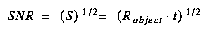where S is the number of detected photons, and Robject is given by the above equations 6.2 through 6.4, and t is the exposure time.

In the background limited case (e.g. read noise, dark current, or sky noise limited) the SNR is a function not only of the expected number of detected photons S from the source but also of the average effective background count rate in each pixel B, the point spread function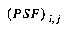, and the weights used to average the signal in the pixels affected by the source. It is easy to show that the signal-to-noise ratio for optimal weights (which are proportional to the point spread function) is given by: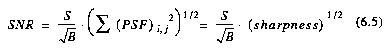Where sharpness is effectively the reciprocal of the number of pixels contributing background noise. The summation is tabulated for a few representative cases in Table 6.5. To estimate the signal-to-noise, multiply the signal-to-noise obtained, assuming all the flux is in one pixel, by the square root of the value in the Table.

#### Table 6.5: Sharpness as a Function of Wavelength.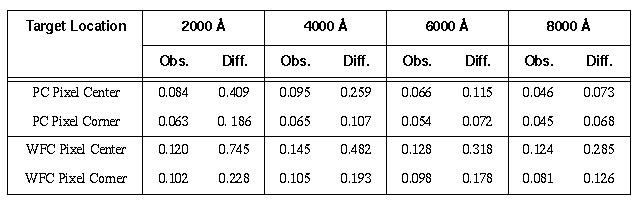We note that PSF fitting is equivalent to convolving the image with the PSF, and then measuring the peak counts for stellar objects. Also, the location of the star on the pixel grid will be impossible to know in advance of the observation (i.e. pixel center vs. pixel corner in Table 6.5). In general, the lower "pixel corner" values should be used, so as to insure adequate SNR.

The average effective background counts per exposure and per pixel can be expanded to include various sources: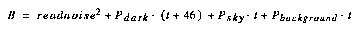For example, Table 2.2 lists the faintest V magnitude star, V=28.14, measurable with a signal-to-noise ratio of 3 in a 3000s integration in F569W in the Wide Field Cameras. The calculation to check this goes as follows. The efficiency of the filter is 0.02139 from Table 6.1. The sky background in each pixel is 23.3+5=28.3, assuming an ecliptic latitude of 90 degrees from Table 6.3, and the pixel area correction for the WFC given in that section. The total sky background collected per pixel in 3000 seconds is given by Equation 6.1 as 76.8 electrons. Note that the AB_n color correction required for the sky in the wavelength range of the filter is 0.0 from Table 6.2. From Table 4.3, the read noise is 5.2 electrons. From Table 4.2, the median dark current at -88 degrees C is 0.004. Therefore the total dark current (on which there will be shot noise) is only 12 electrons. The equivalent background per pixel is then given as B=76.8+5.22+12=116. The total number of detected electrons from a star with V=28.14 is S=89 electrons, again using Equation 6.1. The expected peak count is 27 detected electrons using Table 5.4, which is much less than B, requiring the use of Equation 6.5 for the background limited case. The sharpness for the WF camera in the best case, when the star is centered on a pixel, is given in Table 6.5 as 0.128. Then Equation 6.5 above gives the signal-to-noise as 3.0: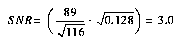If, instead, the peak count rate comes out much greater than the background, the observation is photon noise limited, and the signal-to-noise should be computed as the square root of the signal S in electrons.

In principle, one should also include contributions in the signal-to-noise for flat fielding uncertainties, noise in the bias and dark calibration files, and quantization noise. Flat fielding errors will be of order 1%, and will limit SNR in the large-signal limit. Noise in the bias and dark calibration files will be unimportant in most pixels, although these could become important if many (>10) non-dithered frames of the same field are combined.

A generalized equation for estimating point source signal-to-noise ratio per exposure is given below (Equation 6.6). It is exact in both the bright and faint object limits, and is a reasonable approximation to the intermediate case. Pbackground represents any generalized source of diffuse background light (e.g. galaxy on which target is superposed). Table 6.6 gives rough values for some of the parameters, along with references for more accurate values.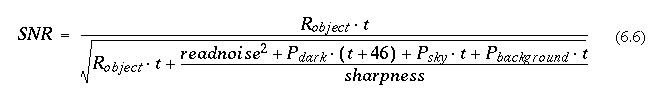Note that in this formulation, sharpness-1 is the equivalent number of pixels the weighted signal is integrated over. In the event that multiple exposures are taken (e.g. to remove cosmic rays), the signal-to-noise ratio for the final averaged image is approximately given by: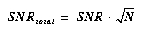where N is the number of images averaged.

#### Table 6.6: Parameters for Point Source SNR Estimation - PSF Fitting.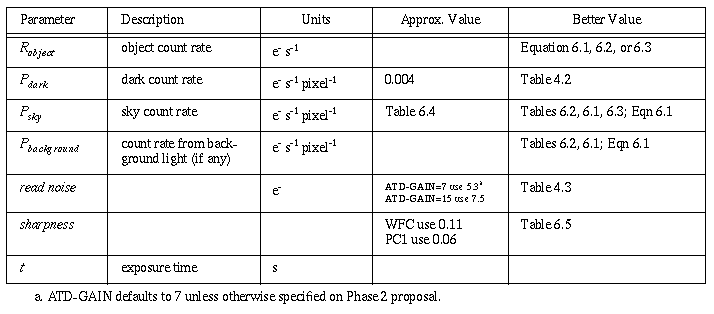## 6.5.2 Point Sources -- Aperture Photometry

When aperture photometry is used, one must consider the fraction of the object counts encircled by the aperture, as well the background noise in the aperture. In the bright target limit the SNR is given by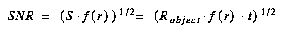where S is the number of detected photons, f(r) is the fraction of the total counts encircled by the aperture with radius r, and Robject is target count rate. Representative values of f(r) are given in Table 6.7; values for other aperture sizes and filters can be estimated from Figure 5.3 on page 88, or Figure 5.4 on page 89.

In the faint target limit the noise contributed by background counts determines the SNR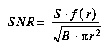where B represents the effective background counts per pixel, and r is the aperture radius in pixels.

In the generalized case the SNR per exposure for aperture photometry is given approximately by: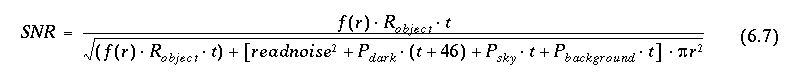where the parameters are summarized in Table 6.8.

#### Table 6.7: Encircled Energy for Representative Filters.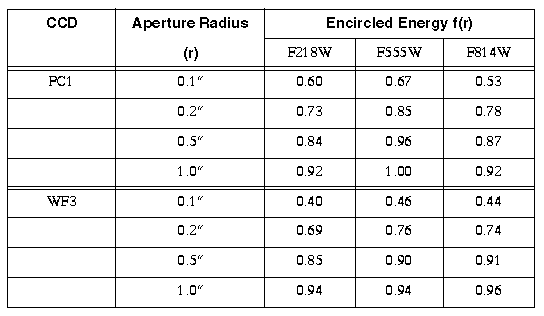#### Table 6.8: Parameters for Point Source SNR Estimation - Aperture Photometry.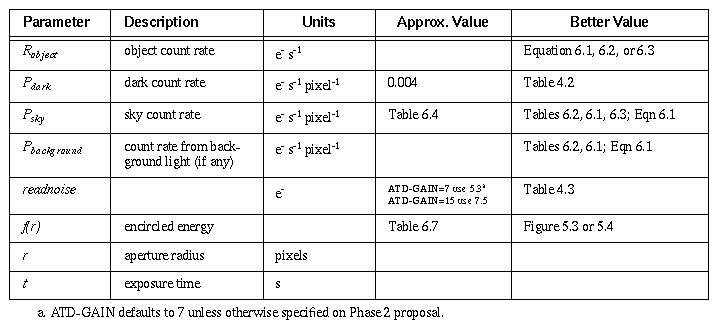## 6.5.3 Extended Sources

The calculations for extended sources are nearly identical to those for point sources. The easiest procedure is to compute the SNR per detector pixel, and then adjust this value if the total SNR is required for an area encompassing many pixels.

In general, one will have the target magnitude or flux per square arcsecond. To compute the flux per pixel for the PC one merely multiplies the flux per square arcsecond by 0.00207, or instead, adds the value 6.7 to the magnitude per square arcsecond to get the necessary magnitude per PC pixel. For the WFC, one either multiplies the flux per square arcsecond by 0.00993, or adds 5.0 to the magnitude per square arcsecond. Equations 6.2, 6.3, and 6.4 can be rewritten including these factors as below.

### PC Camera

For the PC camera, sources with stellar spectra, and V surface brightness per square arcsecond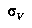we have a count rate in e- s-1 pixel-1 of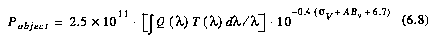For power law sources where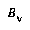is the target flux in units of ergs cm-2 s-1 Hz-1 arcsec-2 we have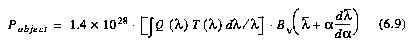And finally for emission line sources where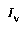is the flux in ergs cm-2 s-1 arcsec-2 we have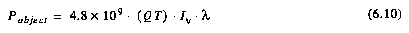where the emission line wavelength l is in Angstroms.

### WFC Camera

For the WFC camera and stellar sources with V surface brightness per square arcsecondwe have a count rate in e- s-1 pixel-1 of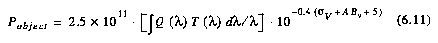For power law sources whereis the target flux in units of ergs cm-2 s-1 Hz-1 arcsec-2 we have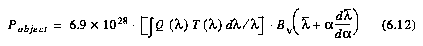And finally for emission line sources whereis the flux in ergs cm-2 s-1 arcsec-2 we have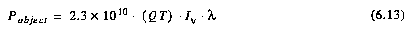where the emission line wavelength l is in Angstroms.

### SNR

The generalized SNR per pixel per exposure for an extended source is then obtained simply by setting the sharpness to unity in equation 6.5: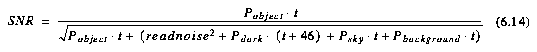#### Table 6.9: Parameters for Extended Source SNR Estimation.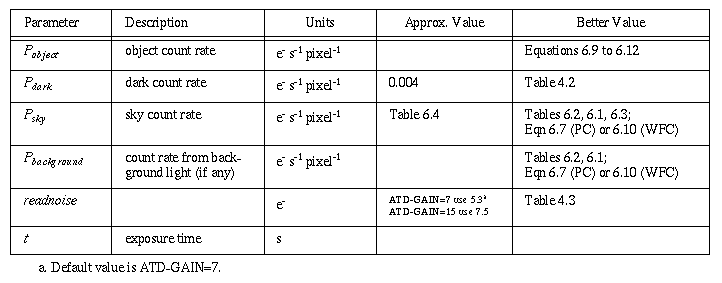For galaxies of very small angular size at red-shifts of cosmological interest, the image may cover a small number of pixels; thus the detection of such objects follows rules similar to those of point sources. However, the fraction of light falling in the central pixel is smaller for most galaxies than it is for true point sources. The approximate magnitude difference between the light falling in the central pixel and the entire galaxy is plotted in Figure 6.1 for a typical giant elliptical galaxy, as a function of redshift. For other types of galaxies, a morphological term can be added to the values (for example, 0.6 magnitudes for lenticulars, 0.7 for S, 0.8 for Sab, 0.9 for Sbc, 1.2 for Scd, and 1.8 for Irr). These values must be increased by an additional 1.7 magnitudes for the PC.

#### Figure 6.1: Giant Elliptical Galaxy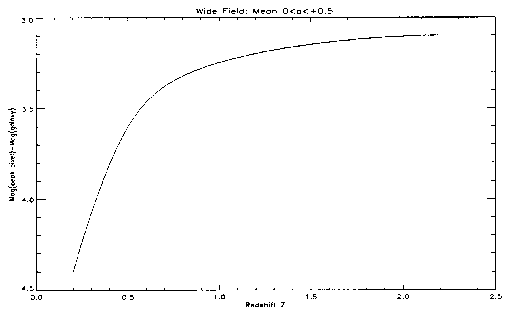6.5.1 - Point Sources -- PSF Fitting
6.5.2 - Point Sources -- Aperture Photometry
6.5.3 - Extended Sources
PC Camera
WFC Camera
SNR
Figure 6.1: - Giant Elliptical Galaxy